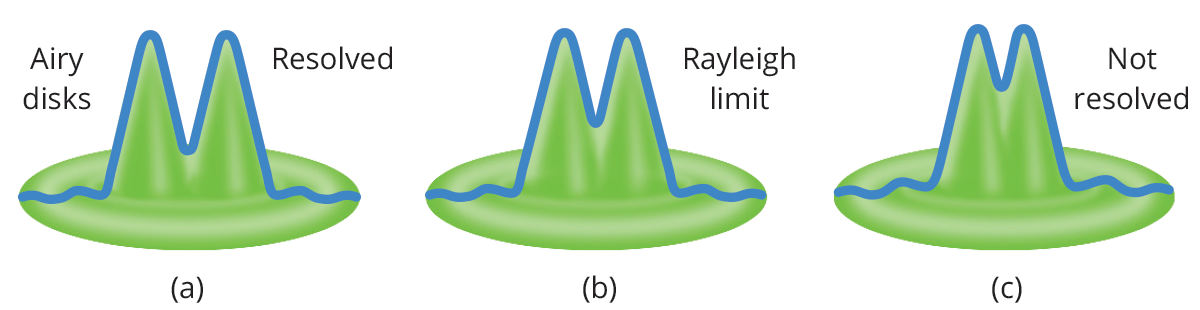# Optics and sensor resolution

The cutoff spatial frequency is not an interesting parameter since machine vision systems cannot reliably resolve features with very low contrast. It is thus convenient to choose a limit frequency corresponding to 20% contrast.

A commonly accepted criterion to describe optical resolution is the Rayleigh criterion, which is connected to the concept of resolution limit. When a wave encounters an obstacle - e.g. it passes through an aperture - diffraction occurs. Diffraction in optics is a physical consequence of the wave-like nature of light, resulting in interference effects that modify the intensity pattern of the incoming wavefront.

Since every lens is characterized by an aperture stop, the image quality will be affected by diffraction, depending on the lens aperture: a dot-like object will be correctly imaged on the sensor until its image reaches a limit size; anything smaller will appear to have the same image – a disk with a certain diameter depending on the lens F/# and on the light wavelength.

This circular area is called the Airy disk, having a radius of

r_A = 1.22 λ f / d

where λ is the light wavelength, f is the lens focal length, d is the aperture diameter and f /d is the lens F-number. This also applies to distant objects that appear to be small.

If we consider two neighboring objects, their relative distance can be considered the “object” that is subject to diffraction when it is imaged by the lens. The idea is that the diffraction of both objects’ images increases to the point that it is no longer possible to see them as separate. As an example, we could calculate the theoretical distance at which human eyes cannot distinguish that a car’s lights are separated. The Rayleigh criterion states that two objects are not distinguishable when the peaks of their diffraction patterns are closer than the radius of the Airy Disk rA (in image space).

The Opto Engineering® TC12120 telecentric lens, for example, will not distinguish feature closer thanr_A = 1.22 * 0.587 µm * 8 = 5.7 µm

in image space (e.g. on the sensor). The minimum resolvable size in image space is always 2 rA, regardless of the real-world size of the object. Since the TC12120 lens has 0.052X magnification and 2rA = 11.4 µm, the minimum real-world size of the object that can be resolved is 11.4 µm /0.052 = 220 µm.

For this reason, optics should be properly matched to the sensor and vice versa: in the previous example, there is no advantage to use a camera with 2 µm pixel size, since every “dot-like” object will always cover more than one pixel. In this case, a higher resolution lens or a different sensor (with larger pixels) should be chosen. On the other hand, a system can be limited by the pixel size, where the optics would be able to “see” much smaller features.

The Transfer Function of the whole system should then be considered, assessing the contribution from both the optics and the sensor. It is important to remember that the actual resolution limit is not only given by the lens F/# and the wavelength, but also depends on the lens aberrations: hence, the real spatial frequency to be taken into account is the one described by the MTF curves of the desired lens.

Next →